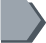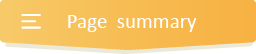Your browser does not support JavaScript!

#Dividing fractions practice for 6th Grade with answers### What is the keep-change-flip method for dividing fractions?

Engage and have a daily practice with this fun resource and get to know what is the keep-change-flip method for dividing fractions. We must recall that when given a fraction and a whole number to divide or multiply, we must change that whole number to a fraction by putting the number over a denominator of 1.

So when you have two fractions (proper or improper) to divide, use the keep-change-flip method, which means that;

• KEEP = Keep the first fraction as it is and leave it alone.

• CHANGE = Change the division sign to a multiplication sign.

• FLIP = Flip the second fraction (swap the numerator and the denominator)

You can now solve the problem by multiplying the fractions and simplifying the answer if necessary.

You should note that when dividing mixed numbers, you must begin by converting the mixed numbers into improper fractions before solving them.

•